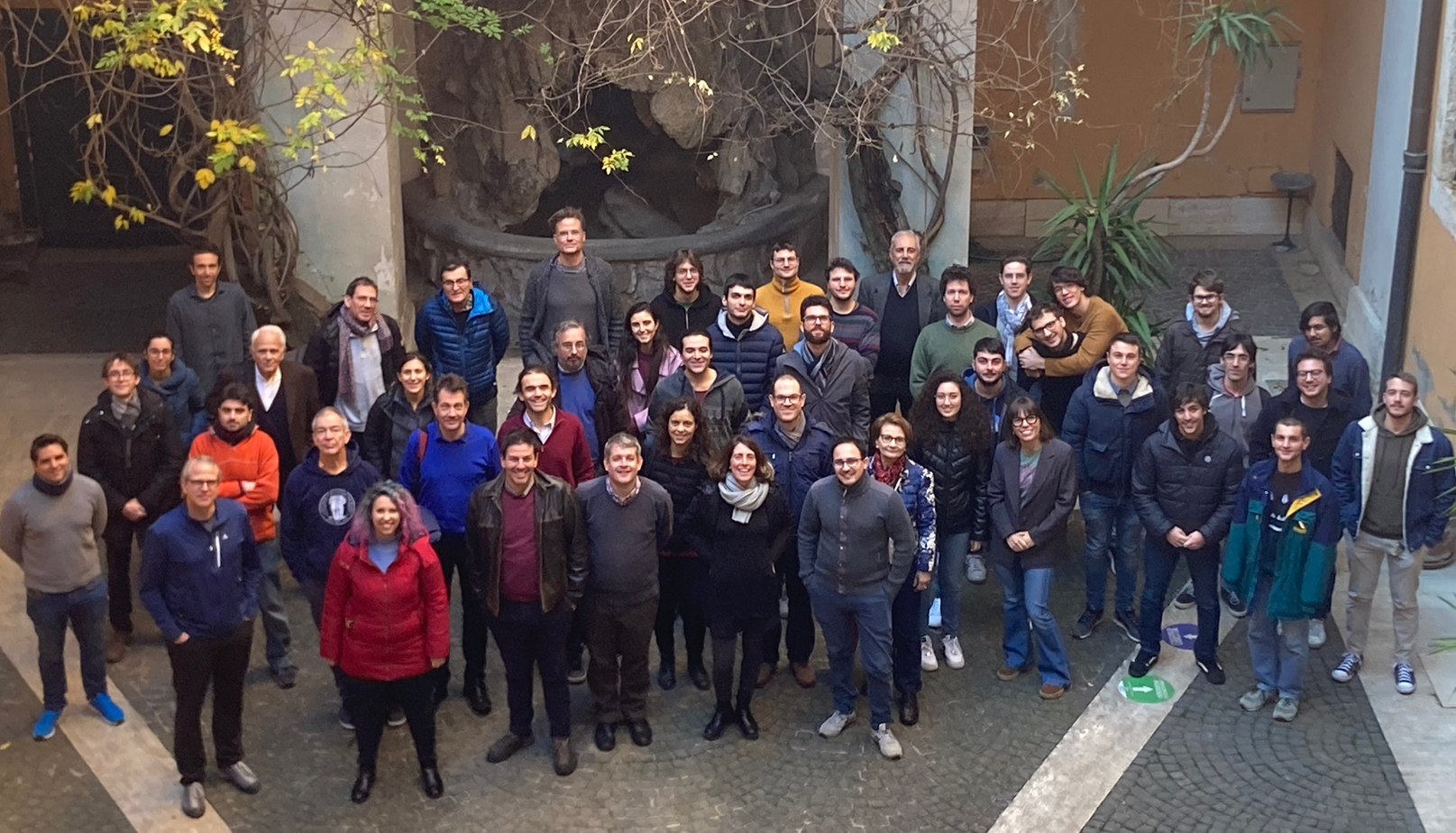## Winter Meeting in Algebra and Geometry

Rome : December 19 - 21 2022## Speakers

Name Institution
Lucia Caporaso Roma Tre
Giuseppe Pareschi Tor Vergata
Federico Pellarin Sapienza
Giulia Saccà Columbia University
Luca Schaffler Roma Tre
René Schoof Tor Vergata
Jakob Stix Goethe University Frankfurt
Catharina Stroppel University of Bonn
Daniele Valeri Sapienza
Alessandro Verra Roma Tre

## Venue

Aula Urbano VIII dell’Argiletum (Università Roma Tre, Dipartimento di Architettura), Via della Madonna dei Monti, 40 – 00184 Roma

History. Since 2011, the Algebra and Geometry research groups of the three main universities in Rome (Sapienza, Tor Vergata and Roma Tre University) have undertaken the tradition of organizing a winter meeting to bring together local and world-wide researchers and to foster collaborations and exchange of ideas. Each time, a variety of topics in Algebra and Geometry is selected to be showcased.

Previous Editions: 2021, 2019 and 2018.

## Abstracts

Abstract: The structure of the moduli spaces of curves has been under investigation for a long time, from several perspectives, including arithmetic and topology. This expository talk will describe some recent progress, mostly on its topology, relating to less recent discoveries from the late 1900.

Abstract: I will talk about a joint work with Federico Caucci and Luigi Lombardi on various derived invariants of smooth complex projective varieties governed by the Albanese map. These include the relative canonical ring and the class of the relative canonical model in suitable variants of the Grothendieck ring of varieties, with application to the derived invariance of Hodge numbers.

Abstract: Drinfeld modular forms can be viewed as analogues in equal positive characteristic global function fields of classical modular forms. Introduced by D. Goss in his 1977 thesis, they are currently studied by several authors. The analogy with classical modular forms is somehow superficial as, for example, while they are submitted to predictable structural algebraic properties, they carry a completely different and in any case not well understood connection with the theory of L-functions. In this talk we shall present some results where we try to interpret in a larger picture the existence of analytic families of such forms at the place infinity. As far as we can see, these families have no analogues in the usual theory. We will explain how certain such families (Eisenstein families) can be used to detect functional identities for avatars of zeta functions.

Abstract: If $$X$$ is a smooth projective variety of dimension $$n$$ and $$L$$ is the class of an ample line bundle on $$X$$, the Hard Lefschetz Theorem says that for every $$k=0,\dots,n$$, the cup product map $$L^{n-k}: H^k(X) \to H^{2n-k}(X)$$ is an isomorphism. The Lefschetz Standard conjecture, proposed by Grothendieck in 1968, predicts that the inverse of this isomorphism is induced by an algebraic cycle on $$X \times X$$. In this talk I will present some recent results proving the Lefschetz Standard conjecture for certain Lagrangian fibered hyper-Kähler manifolds. This is joint work with G. Ancona, M. Cavicchi, and R. Laterveer.

Abstract: Smooth minimal surfaces of general type with $$K^2=1, p_g=2$$, and $$q=0$$ constitute a fundamental example in the geography of algebraic surfaces, and the 28-dimensional moduli space $$\mathbf{M}$$ of their canonical models admits a modular compactification $$\overline{\mathbf{M}}$$ via the minimal model program. We describe eight new irreducible boundary divisors in such compactification parametrizing reducible stable surfaces. Additionally, we study the relation with the GIT compactification of $$\mathbf{M}$$ and the Hodge theory of the degenerate surfaces that the eight divisors parametrize. This is joint work with Patricio Gallardo, Gregory Pearlstein, and Zheng Zhang.

Abstract: In this expository talk we discuss Galois representations associated to elliptic curves over number fields. Starting with Serre's paper of the 1970'ies we describe the successive work of Mazur, Bilu, Parent, Rebolledo, Balakrishna et al. and the unsolved problems involving Serre's Uniformity Conjecture.

Abstract: Abelian varieties over finite fields are described up to isogeny by Weil numbers. We will recall this Honda-Tate theory and explain how to raise the description to a categorical level in terms of categories of modules. This extends and reproves prior equivalences of categories by Deligne for ordinary abelian varieties to the case of all abelian varieties over finite fields. A prominent role in the theory is played by what we call locally projective abelian varieties. This is joint work with Tommaso Centeleghe.

Abstract: In this Talk I will explain a geometric approach towards describing Ext algebras of standard objects in highest weight categories. As a special example we will look at the case of perverse sheaves on Grassmannians and provide a geometric, combinatorial and algebraic description.

Abstract: W-algebras are families of algebraic structures at the crossroad of representation theory and mathematical physics. In the "affine" case, they can be regarded as a generalization of affine Kac-Moody algebras and the Virasoro algebra, and appeared in the study of two dimensional rational Conformal Field Theories. Their "finite" analogue comes from (geometric) representation theory: just as the universal enveloping algebra is a quantization of $$\mathfrak{g}^{**}$$, the dual of a finite dimensional Lie algebra $$\mathfrak{g}$$, with its Kostant-Kirillov Poisson bracket, the finite W-algebra is a quantization of an affine subspace of $$\mathfrak{g}^{**}$$, obtained via Hamiltonian reduction, called the Slodowy slice. In the talk, we review basic facts about W-algebras and the construction of Lax type operators for finite W-algebras. These operators are used to investigate the relationship between (twisted) Yangians and finite W-algebras.

Abstract: After an Introduction to the themes relating Enriques surfaces and Rationality questions, the Artin-Mumford counterexample to the Luroth problem is revisited. A construction of it is given, which is explicitly related to the geometry of Enriques surfaces, more precisely to the special family of Reye congruences and their classical geometry. The same construction extends to higher dimensions.

## Schedule

Time Monday 19 Tuesday 20 Wednesday 21
9:30 - 10:30 Saccá Pellarin
10:30 - 11:00 Break
11:00 - 12:00 Schaffler Caporaso
12:00 - 14:00 Lunch Break
14:00 - 15:00 Stroppel Schoof
15:00 - 16:00 Verra Valeri
16:00 - 16:30 Break
16:30 - 17:30 Stix Pareschi